# Types of Vectors and Their Definitions in Physics – Scalars and Vectors

Types of Vectors
(i) Equal Vectors:
Two vectors of equal magnitude and having same direction are called equal vectors.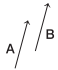We are giving a detailed and clear sheet on all Physics Notes that are very useful to understand the Basic Physics Concepts.

## Types of Vectors and Their Definitions in Physics – Scalars and Vectors

(ii) Negative Vectors:
Two vectors of equal magnitude but having opposite directions are called negative vectors.
The negative vector of A is represented as -A.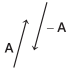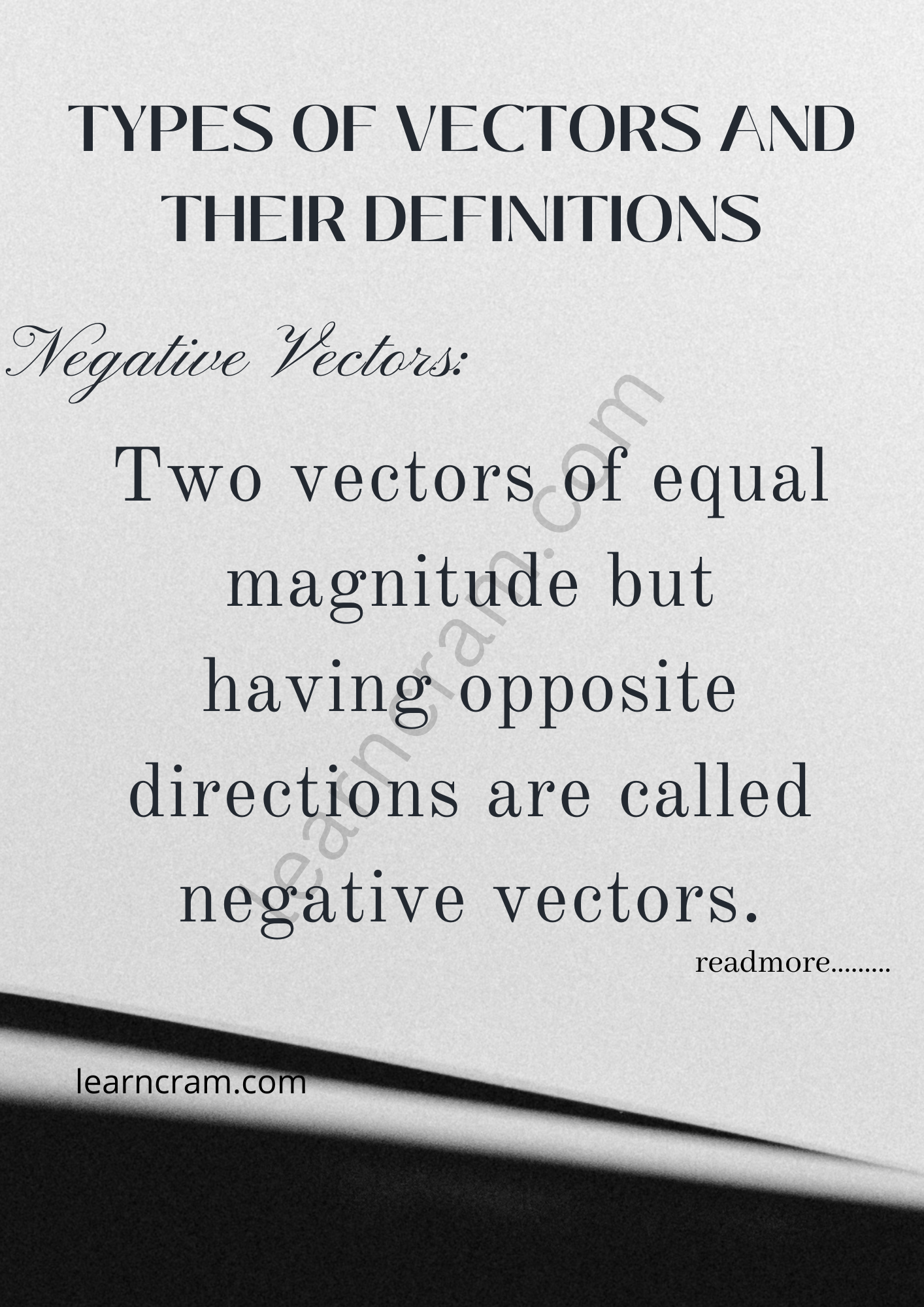(iii) Zero Vector or Null Vector:
A vector whose magnitude is zero, known as a zero or null vector. Its direction is not defined. It is denoted by 0.

Velocity of a stationary object, acceleration of an object moving with uniform velocity and resultant of two equal and opposite vectors are the examples of null vector.

(iv) Unit Vector:
A vector having unit magnitude is called a unit vector.
A unit vector in the direction of vector A is given by
$$\hat{\mathbf{A}}=\frac{\mathbf{A}}{A}$$
A unit vector is unitless and dimensionless vector and represents direction only.

(v) Orthogonal Unit Vectors:
The unit vectors along the direction of orthogonal axis, i.e. X-axis, Y-axis and X-axis are called orthogonal unit vectors. They are represented by $$\hat{\mathbf{i}}, \hat{\mathbf{j}} \text { and } \hat{\mathbf{k}}$$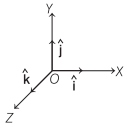(vi) Co-initial Vectors Vectors:
having a common initial point, are called co-initial vectors.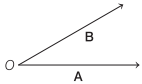(vii) Collinear Vectors:
Vectors having equal or unequal magnitudes but acting along the same or parallel lines are called collinear vectors.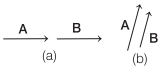(viii) Coplanar Vectors:
Vectors acting in the same plane are called coplanar vectors.

(ix) Localised Vector:
A vector whose initial point is fixed, is called a localised vector.

(x) Non-localised or Free Vector:
A vector whose initial point is not fixed is called a non-localised or a free vector.

(xi) Position Vector:
A vector which gives position of an object with reference to the origin of a coordinate system is called position vector. It is represented by a symbol r.(xii) Displacement Vector: The vector which tells how much and in which direction an object has changed its position in a given interval of time is called displacement vector.

Displacement vector is the straight line joining the initial and final positions and does not depend on the actual path undertaken by the object between the two positions.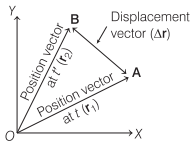The displacement vector for AB is
Δr = r2 – r1

Scalars and Vectors Topics: